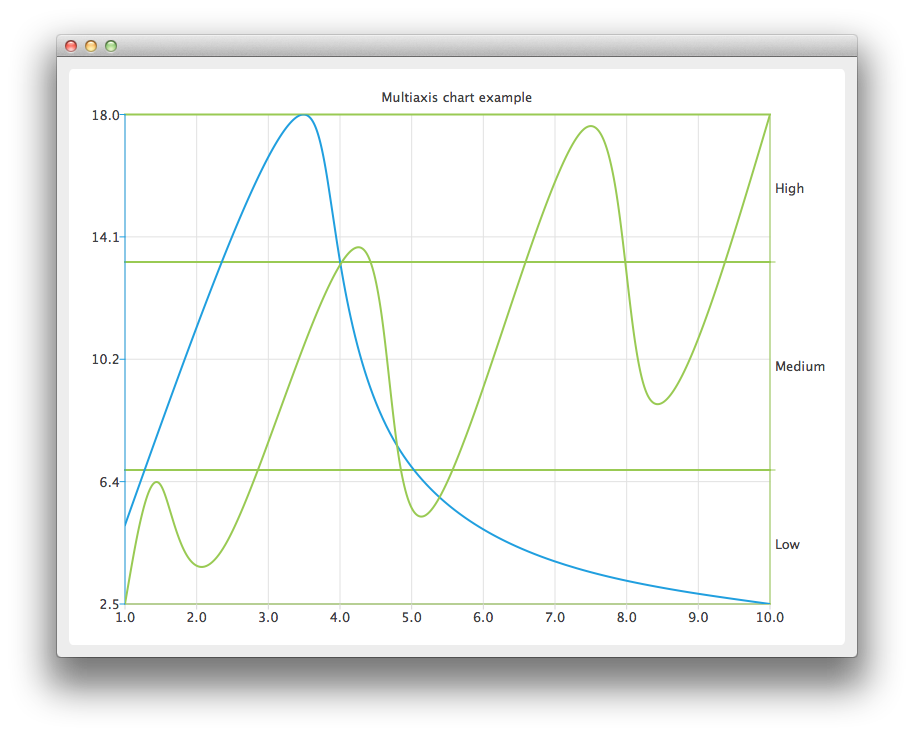# Multiple Axes Example

The example shows how to create a simple chart with two vertical axes. One for each series.## Running the Example

To run the example from Qt Creator, open the Welcome mode and select the example from Examples. For more information, visit Building and Running an Example.

## Using Multiple Axes

Create the QChart instance, hide the legend of the chart, and set its title.

```QChart *chart = new QChart();
chart->legend()->hide();
chart->setTitle("Multiaxis chart example");```

Create a QValueAxis instance that will be used as a horizontal axis by both series and add it the bottom of the chart. The axis can be shared between many series, but each series can have only one vertical and horizontal axis.

```QValueAxis *axisX = new QValueAxis;
axisX->setTickCount(10);

Create the first series, and add the data to it. Finally, add the series to the chart. Instantiate its own Y-axis, add it to the chart. Then attach both the common X-axis and the series specific Y-axis. In this example the color of the axis line is set to be the same as the color of the series to make it possible to distinguish which axis is attached to which series.

```QSplineSeries *series = new QSplineSeries;
*series << QPointF(1, 5) << QPointF(3.5, 18) << QPointF(4.8, 7.5) << QPointF(10, 2.5);

QValueAxis *axisY = new QValueAxis;
axisY->setLinePenColor(series->pen().color());

series->attachAxis(axisX);
series->attachAxis(axisY);```

Similarly prepare another series. This time a different axis type is used. Additionally grid lines color is also set to be the same as the color of the series.

```series = new QSplineSeries;
*series << QPointF(1, 0.5) << QPointF(1.5, 4.5) << QPointF(2.4, 2.5) << QPointF(4.3, 12.5)
<< QPointF(5.2, 3.5) << QPointF(7.4, 16.5) << QPointF(8.3, 7.5) << QPointF(10, 17);

QCategoryAxis *axisY3 = new QCategoryAxis;
axisY3->append("Low", 5);
axisY3->append("Medium", 12);
axisY3->append("High", 17);
axisY3->setLinePenColor(series->pen().color());
axisY3->setGridLinePen((series->pen()));

series->attachAxis(axisX);
series->attachAxis(axisY3);```

Create a QChartView object with QChart as a parameter. Enable Antialiasing to have the rendered splines look nicer.

```QChartView *chartView = new QChartView(chart);
chartView->setRenderHint(QPainter::Antialiasing);```

The chart is ready to be shown.

```QMainWindow window;
window.setCentralWidget(chartView);
window.resize(800, 600);
window.show();```

Files: